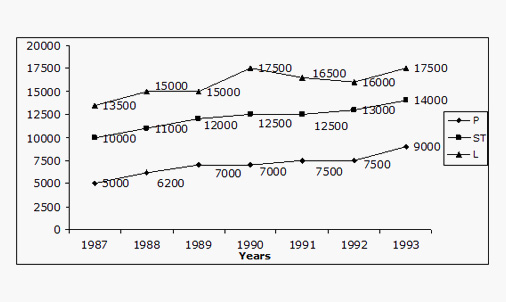1. Math
2. Mathematics
3. refer to the graph given below and answer the questions...

# Question: refer to the graph given below and answer the questions...

###### Question details

Refer to the graph given below and answer the questions given after that accordingly.
The graph given shows price variation of three types of wood over 1987 – 1993 in Rs/m3. 1 ton = 1000 kg and 1m3 = 800 kg.Ex.9 Maximum increase in price/ m3 for any product for any two consecutive years was in which of the products?
1. P
2. ST
3. L
4. None of these

Ex.10 In 1993, a company's total sales was made of 40% of P, 30% of ST and 30% of L. The average realization per m3 in 1993 was:
1. Rs. 8121
2. Rs. 7211
3. Rs. 6567
4. None of these

###### Solution by an expert tutor